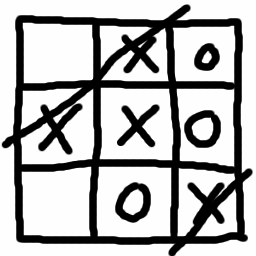# Games, Geometry and Logic## Introduction

These materials are designed for high school students as an introduction to some current ideas in geometry and logic through the use of two-player strategy games. Each unit below gives some new games to play, along with questions and new mathematical ideas which lead to better winning strategies. Some of these units (such as Hypergame) may fit in a single period, while others (such as Games as Trees) would be better off spread over two or three sessions. In these cases, the students should be encouraged to play the game against each other and develop strategies, while the later classes would introduce the mathematical abstractions that allow us to solve these games more completely.
Each page ends with a link to several other websites either containing more information on the games, more information on the mathematical topics introduced, or games which are similar to the ones given.
The units are somewhat independent, but occasionally draw on concepts developed in earlier units. In particular, the notion of a game tree is used in several of the questions in Games as Numbers.

## Lessons

Typical Games on Atypical Surfaces, an introduction to the topology of surfaces via board games.
Brussels Sprouts and the Euler Characteristic, using topological invariants to predict the winner of a game.
Games as Trees, creating an abstract notion of "game" and computing the winner of any two-player game automatically.
Games as Numbers, taking Games as Trees one step farther by creating a whole arithmetic of games.